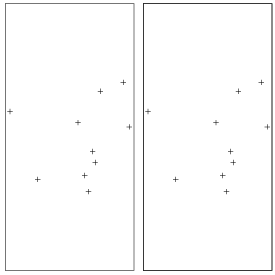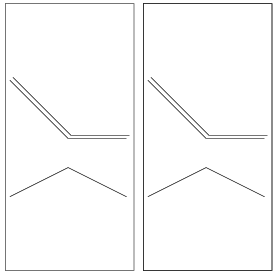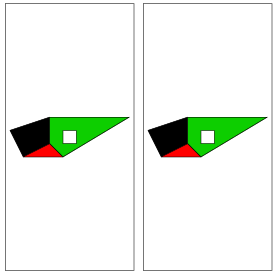## Working with sp and aRT

July 20, 2009

### 1 Introduction

sp (Pebesma & Bivand, 2005) is an important package that defines standards and allows for exchanging information between spatial packages in R. As aRT manipulates all spatial data formats, it was designed to follow sp standards to represent spatial data, storing and retrieving data using the sp format. Therefore, when working with aRT and spatial data in R it is required that objects containing spatial data are converted, whenever necessary, to the sp format. For futher details on sp package see http://r-spatial.sourceforge.net.

On the other hand, TerraLib databases can contain data that cannot be directly converted to sp data. For example:

1. TerraLib (and therefore aRT) requires ID in all spatial data, different from sp, that requires ID only for lines and polygons.
2. TerraLib layers have support to multigeometry, meaning that each spatial element can have more than one geometry associated. For example, a layer of cities can store both contours and centroids.
3. geometries and attributes are stored in different objects in a TerraLib database. Geometries are stored directly inside layers, whereas attributes are stored in tables inside layers. The reason why tables cannot be in the same object as geometries is because TerraLib supports different table formats, for example static, event, and dynamic.

This document illustrates how to manipulate spatial data in aRT, showing how to import to and read from TerraLib databases. The data (and also some sentences!) of this document are extracted from Pebesma and Bivand (2005).

We start by loading the package and, for convenience here, setting aRT to the silent mode which hides some information messages issued by aRT functions; followed by establishing a connection to a DBMS. The database to be used in the examples is called “sp”, which, if exists, is removed from the DBMS and recreated. Notice this is a TerraLibdatabase, i.e., a database created following the TerraLib structure.

> require(aRT)
> aRTsilent(TRUE) # hiding info messages

 TRUE

> con = openConn(name="default")
> if(any(showDbs(con)=="sp")) deleteDb(con, "sp", force=T)
> db = createDb(con, "sp")

Further details on connections to the DBMS are provided by the vignette aRTconn.

### 2 Spatial points

#### 2.1 Points without attributes

Our first example illustrates how to handle data with a points geometry. For instance, consider a set of 10 points randomly generated on the unit square [0,1] × [0,1] and stored in a matrix xy. The first step is to use sp to convert into a SpatialPoints object.

> xy = matrix(runif(20), nc=2)
> xy.sp = SpatialPoints(xy)
> xy.sp

SpatialPoints:
coords.x1 coords.x2
[1,] 0.6739453 0.3021973
[2,] 0.9619853 0.6170330
[3,] 0.9228386 0.9050982
[4,] 0.7747456 0.8476483
[5,] 0.7248062 0.4579902
[6,] 0.7413480 0.3863993
[7,] 0.6302933 0.6443511
[8,] 0.1903071 0.7170724
[9,] 0.6977443 0.1980982
[10,] 0.3703263 0.2772779
Coordinate Reference System (CRS) arguments: NA

However, an SpatialPoints object cannot be used by aRT functions because it does not have ID and it is necessary to convert to a SpatialPointsDataFrame.

> xy.spdf = SpatialPointsDataFrame(xy, data.frame(ID=paste(1:10)))

Note that here you can use both xy or xy.spdf as argument to Spatial PointsDataFrame.

The first step to store this data in a TerraLib database is to create a layer. A layer is a container that can store any geometric type and also, optionally, other types of objects. A layer can be created in a database using createLayer() and the function call receives as arguments the database object and a string defining the name to be set for the layer.

> lpoints = createLayer(db, "points")
> lpoints

Object of class aRTlayer

Layer: "points"
Database: "sp"
Layer is empty
Projection Name: "NoProjection"
Projection Datum: "Spherical"
Tables: (none)

Notice that we have two names, "points" which is the name of the layer in the database, and lpoints, an R object which can access "points". Next the function addPoints() is used to store the points into the layer. Notice that after that the layer object acknowledges the points are now stored into the database.

> lpoints

Object of class aRTlayer

Layer: "points"
Database: "sp"
Number of points: 10
Projection Name: "NoProjection"
Projection Datum: "Spherical"
Tables: (none)

To conclude storing the points a further step creating adding a table to the layer is still necessary in order to ba able to read any data from the layer. This is a TerraLib requirement needed even when the spatial data does not have any attributes. Geometries with no entry in any table cannot be retrieved from the database.

> tpoints = createTable(lpoints, "tpoints")
> tpoints

Object of class aRTtable

Table: "tpoints"
Type: static
Layer: "points"
Rows: 10
Attributes:
id: character (key)

> lpoints

Object of class aRTlayer

Layer: "points"
Database: "sp"
Number of points: 10
Projection Name: "NoProjection"
Projection Datum: "Spherical"
Tables:
"tpoints": static

Now the layer has 10 points and one table, and we can retrieve the point coordinates using getPoints(), which returns an object of class SpatialPointsDataFrame:

> points = getPoints(lpoints)
> points

coordinates ID
1  (0.673945, 0.302197)  1
2  (0.370326, 0.277278) 10
3  (0.961985, 0.617033)  2
4  (0.922839, 0.905098)  3
5  (0.774746, 0.847648)  4
6   (0.724806, 0.45799)  5
7  (0.741348, 0.386399)  6
8  (0.630293, 0.644351)  7
9  (0.190307, 0.717072)  8
10 (0.697744, 0.198098)  9

Note that the points have a different order from the original data. That is because the database stores the IDs as characters, therefore 10 comes before 2.

Figure 2 illustrates two different ways for visualising the point coordinates, on the left by plotting the points from an R object with plot(points), or directly from the layer, with plot(lpoints), which does not requires the existence of an R object containing the points.Figure 1: Plot of a layer with points

#### 2.2 Points with attributes

Another possible way of creating a SpatialPointsDataFrame object is by building it by combining a SpatialPoints object and a data frame containing associated attributes. In the following example we combine the object xy.sp with a data frame df containg attributes. Notice the ID column is required by any sp object.

> df = data.frame(z1 = round(5 + rnorm(10), 2), z2 = 0:9, ID = paste(1:10))
> xy.spdf = SpatialPointsDataFrame(xy.sp, df)
> xy.spdf

coordinates   z1 z2 ID
1  (0.673945, 0.302197) 3.10  0  1
2  (0.961985, 0.617033) 4.15  1  2
3  (0.922839, 0.905098) 3.68  2  3
4  (0.774746, 0.847648) 4.45  3  4
5   (0.724806, 0.45799) 6.62  4  5
6  (0.741348, 0.386399) 5.57  5  6
7  (0.630293, 0.644351) 3.66  6  7
8  (0.190307, 0.717072) 3.75  7  8
9  (0.697744, 0.198098) 5.19  8  9
10 (0.370326, 0.277278) 5.02  9 10

As before we create a layer and aad the points to it. Next, as our object now has attributes, we can import the table data using importTable().

> lpointsdf = createLayer(db, "lpointsdf")
> tpointsdf = importTable(lpointsdf, "tpointsdf", ID="ID", xy.spdf)
> tpointsdf

Object of class aRTtable

Table: "tpointsdf"
Type: static
Layer: "lpointsdf"
Rows: 10
Attributes:
id: character (key)
z1: numeric
z2: integer

> lpointsdf

Object of class aRTlayer

Layer: "lpointsdf"
Database: "sp"
Number of points: 10
Projection Name: "NoProjection"
Projection Datum: "Spherical"
Tables:
"tpointsdf": static

When retrieving data from the database to R getting point coordinates and the table at once from the layer we can use a second argument of getPoints() with the table to be read.

> getPoints(lpointsdf, tpointsdf)

coordinates ID   z1 z2
1  (0.673945, 0.302197)  1 3.10  0
2  (0.370326, 0.277278) 10 5.02  9
3  (0.961985, 0.617033)  2 4.15  1
4  (0.922839, 0.905098)  3 3.68  2
5  (0.774746, 0.847648)  4 4.45  3
6   (0.724806, 0.45799)  5 6.62  4
7  (0.741348, 0.386399)  6 5.57  5
8  (0.630293, 0.644351)  7 3.66  6
9  (0.190307, 0.717072)  8 3.75  7
10 (0.697744, 0.198098)  9 5.19  8

#### 2.3 Doing all at once

All the steps above can be encapsulated using importSpData().

### 3 Grids

(not supported yet)

### 4 Lines

#### 4.1 Building line objects from scratch

In many instances, line coordinates will be retrieved from external sources. The following example shows how to build an object of class SpatialLines from scratch. As objects from this class already stores ID, they are pushed in the layer directly using addLines().

> l1 = cbind(c(1,2,3),c(3,2,2))
> l1a = cbind(l1[,1]+.05,l1[,2]+.05)
> l2 = cbind(c(1,2,3),c(1,1.5,1))
> Sl1 = Line(l1)
> Sl1a = Line(l1a)
> Sl2 = Line(l2)
> #S1 = Lines(list(Sl1, Sl1a), ID="a")
> S1 = Lines(list(Sl1), ID="a")
> S2 = Lines(list(Sl2), ID="b")
> S3 = Lines(list(Sl1a), ID="c")
> Sl = SpatialLines(list(S1,S2,S3))
> llines = createLayer(db,"llines")
> createTable(llines, "llines")

Object of class aRTtable

Table: "llines"
Type: static
Layer: "llines"
Rows: 3
Attributes:
id: character (key)Figure 2: Plot of a layer with lines

#### 4.2 Building line objects with attributes

The same as polygons

### 5 Polygons

#### 5.1 Building from scratch

The following example shows how a set of polygons are built from scratch. Note that Sr4 has the opposite direction (right) as the other three; it is meant to represent a hole in the Sr3 polygon.

> #genPol = function(quant)
> #{
> #    res = list()
> #    res = lapply(1:quant, function(x) {
> #        from = round(runif(2,1,20),2)
> #        to   = from + round(runif(2,1,5),2)
> #
> #        Sr1 = Polygon(cbind(c(from,from,to,to,  from),
> #                          c(from,to,  to,from,from)))
> #        Srs1 = Polygons(list(Sr1),paste(x))
> #    })
> #    SR = SpatialPolygons(res, 1:quant)
> #}
>
> Sr1 = Polygon(cbind(c(2,4,4,1,2),c(2,3,5,4,2)))
> Sr2 = Polygon(cbind(c(5,4,2,5),c(2,3,2,2)))
> Sr3 = Polygon(cbind(c(4,4,5,10,4),c(5,3,2,5,5)))
> Sr4 = Polygon(cbind(c(5,6,6,5,5),c(4,4,3,3,4)), hole = TRUE)
> Srs1 = Polygons(list(Sr1), "s1")
> Srs2 = Polygons(list(Sr2), "s2")
> Srs3 = Polygons(list(Sr3, Sr4), "s3/4")
> SR = SpatialPolygons(list(Srs1,Srs2,Srs3), 1:3)
> lrings = createLayer(db, "lrings")
> trings = createTable(lrings, "trings")
> #th=createTheme(lrings, "trings")
> lrings

Object of class aRTlayer

Layer: "lrings"
Database: "sp"
Number of polygons: 4
Projection Name: "NoProjection"
Projection Datum: "Spherical"
Tables:
"trings": static

> #sr = as.SpatialPolygon(getGeometry(lrings))
>
> pols = getPolygons(lrings)Figure 3: plot of a layer with polygons

#### 5.2 Polygons with attributes

Polygons with attributes, objects of class SpatialPolygonsDataFrame, are built from the SpatialPolygons object (topology) and the attributes (data.frame):

> #attr = data.frame(a=1:3, b=3:1, row.names=c("s1","s2","s3/4"))
> #SrDf = SpatialPolygonsDataFrame(SR, attr)
> #lringsdf = createLayer(db, "lringsdf")

To import the attributes, we need to create a table, but, due to the internal differences of sp data storage

> #xy.spdf@data
> #SrDf@data
> #class(SrDf@data)

we need to insert SrDf manually, creating both table and the two integer columns before inserting the data:

> #tringsdf = createTable(lringsdf, "tringsdf", ID="ID", gen=F)
> #createColumn(tringsdf, "a", "i")
> #createColumn(tringsdf, "b", "i")
> #tringsdf
> #lringsdf
>
> #getData(tringsdf)
>
> #sr = as.SpatialPolygons(getGeometry(lringsdf))
> #summary(sr)
> #sr = as.SpatialPolygonsDataFrame(getGeometry(lringsdf))
> #sr

### References

Chambers, J.M., 1998, Programming with data, a guide to the S language. Springer, New York.
Pebesma, E.J. and Bivand, R.S., 2005, Classes and methods for spatial data in R, R-News 5 (2), pp. 9-13.